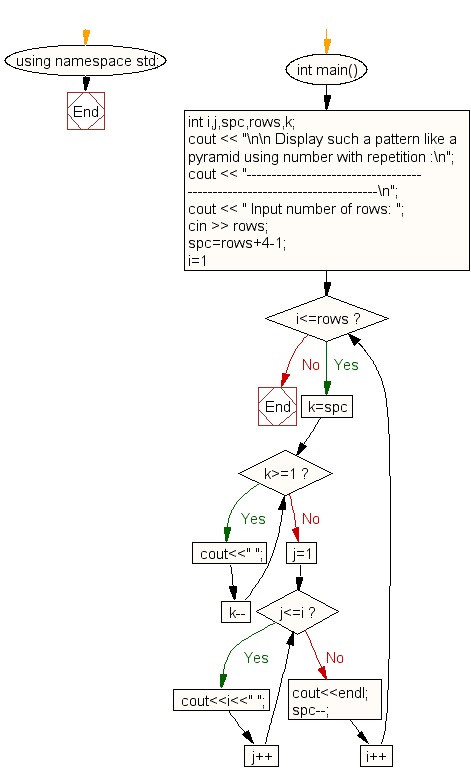﻿ C++ : Print a pyramid pattern with repeating numbers

# C++ Exercises: Display the pattern like a pyramid using number and a number will repeat for a row

## C++ For Loop: Exercise-41 with Solution

Write a C++ program to make such a pattern, like a pyramid, with a repeating number.

Sample Solution:

C++ Code :

``````#include <iostream>
#include <string>
using namespace std;

int main()
{
int i,j,spc,rows,k;
cout << "\n\n Display such a pattern like a pyramid using number with repetition :\n";
cout << "-------------------------------------------------------------------------\n";
cout << " Input number of rows: ";
cin >> rows;
spc=rows+4-1;
for(i=1;i<=rows;i++)
{
for(k=spc;k>=1;k--)
{
cout<<" ";
}
for(j=1;j<=i;j++)
cout<<i<<" ";
cout<<endl;
spc--;
}
}
``````

Sample Output:

``` Display such a pattern like a pyramid using number with repetition :
-------------------------------------------------------------------------
Input number of rows: 5
1
2 2
3 3 3
4 4 4 4
5 5 5 5 5
```

Flowchart:C++ Code Editor: